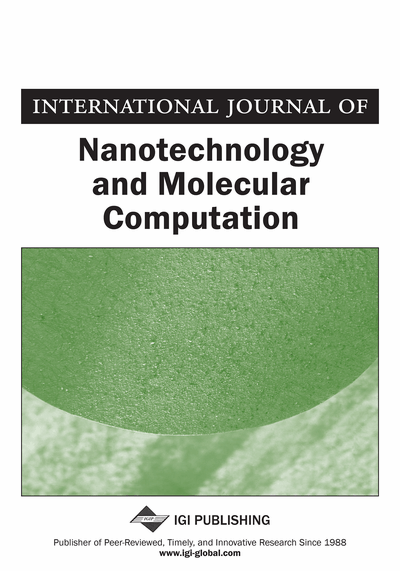# Sequential Voronoi Diagram Calculations using Simple Chemical Reactions

B. P. J. de Lacy Costello, I. Jahan, A. Adamatzky
DOI: 10.4018/ijnmc.2011070103
OnDemand:
(Individual Articles)
Available
\$29.50
No Current Special Offers

## Abstract

In the authors’ recent paper (de Lacy Costello et al., 2010) the authors described the formation of complex tessellations of the plane arising from the various reactions of metal salts with potassium ferricyanide and ferrocyanide loaded gels. In addition to producing colourful tessellations these reactions are naturally computing generalised Voronoi diagrams of the plane. The reactions reported previously were capable of the calculation of three distinct Voronoi diagrams of the plane. As diffusion coupled with a chemical reaction is responsible for the calculation then this is achieved in parallel. Thus an increase in the complexity of the data input does not utilise additional computational resource. Additional benefits of these chemical reactions are that a permanent record of the Voronoi diagram calculation (in the form of precipitate free bisectors) is achieved, so there is no requirement for further processing to extract the calculation results. Previously it was assumed that the permanence of the results was also a potential drawback which limited reusability. This paper presents new data which shows that sequential Voronoi diagram calculations can be performed on the same chemical substrate. This is dependent on the reactivity of the original reagent and the cross reactivity of the secondary reagent with the primary product. The authors present the results from a number of binary combinations of metal salts on both potassium ferricyanide and potassium ferrocyanide substrates. The authors observe three distinct mechanisms whereby secondary sequential Voronoi diagrams can be calculated. In most cases the result was two interpenetrating permanent Voronoi diagrams. This is interesting from the perspective of mapping the capability of unconventional computing substrates. But also in the study of natural pattern formation per se.
Article Preview
Top

## 1. Introduction

Voronoi diagrams are a partitioning of continuous space into regions. The regions are constructed about a finite set of distinct isolated points. Therefore, all locations within the space are associated with the closest member of the point set (Okabe et al., 2009). Thus, Voronoi diagrams have utility as modelling tools where space should be segregated into “spheres of influence.” They find uses in many fields such as astronomy (galaxy cluster analysis (Pastzor & Csillag, 1994)), biology (modelling tumour cell growth (Blackburn & Dunckley, 1996)), chemistry (modelling crystal growth (Hargittai, 1986), ecology (modelling competition (Byers, 1992) and computational geometry (solving nearest neighbor problems (Graham & Yao, 1990). In addition to standard and generalized Voronoi diagrams (where geometric shapes rather than point sources are utilized) weighted diagrams are commonly used, for example, to model crystal growth (Scaudt & Drysdale, 1991).

In addition to their many uses in modelling, Voronoi diagrams can be observed widely in nature, for example, in animal coat markings (Walter et al., 1998) between interacting bacterial, fungal or slime mould colonies (Aurenhammer, 1991; Muller et al., 1998, Shirikawa et al., 2009), between growing crystals (de Lacy Costello et al., 2010) and even in universal structures such as gravitational caustics (Stevens, 1979). They have also been observed and constructed within Gas discharge systems (Zanin et al., 2002 and de Lacy Costello et al., 2004).

The formation of Voronoi diagrams in chemical systems with one reagent and one substrate was first reported by (Tolmachiev & Adamatzky, 1996). This and similar reactions were utilized as chemical processors for the computation of the shortest obstacle free path (Adamatzky & de Lacy Costello, 2003), the skeletonization of a planar shape (Adamatzky & Tolmachiev, 1997; Adamatzky et al., 2002) and the construction of a prototype XOR gate (Adamatzky & de Lacy Costello, 2002). The formation of weighted Voronoi diagrams from two reagents reacted in parallel on one substrate were first reported in (de Lacy Costello, 2003). In the same year the calculation of three Voronoi diagrams in parallel was reported in a chemical system based on the reaction of two reagents on a mixed substrate gel (potassium ferrocyanide and potassium ferricyanide) (de Lacy Costello & Adamatzky, 2003). Complex tessellations of the plane have been constructed in simple chemical reactions where one of two binary reagents exhibited limited or no reactivity with the gel substrate (de Lacy Costello et al., 2009). A more recent paper investigated complex tessellations arising from three reagents on certain substrate loaded gels (de Lacy Costello et al., 2010).

## Complete Article List

Search this Journal:
Reset
Volume 3: 4 Issues (2011)
Volume 2: 4 Issues (2010)
Volume 1: 4 Issues (2009)
View Complete Journal Contents Listing